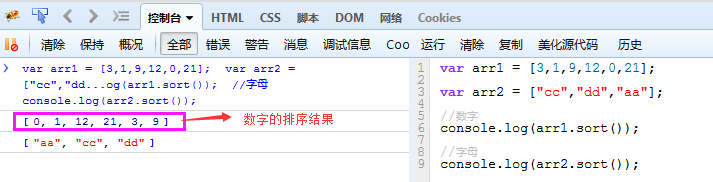# JavaScript对JSON数据进行排序和搜索

## JavaScript实现对象数组按不同字段排序

``````function createComparionFun(propertyName)
{
return function(object1,object2)
{
var value1=object1[propertyName];
var value2=object2[propertyName];
if(value1value2)
{
return 1;
}else {
return 0;
}
}
}
``````

``````var data=[{name:zom,age:18},{name:nbd,age:20}];
data.sort(creatComparionFun(name));
data.sort(creatComparionFun(age));
``````

array_multisort 的用法

\$arr2 = array(6,2,4);
array_multisort(\$arr1,\$arr2);
print_r(\$arr1); // 得到的顺序是1,5,9
print_r(\$arr2); // 得到的顺序是6,4,2

//1、字符串(如”2010-3-1″格式)转换成Date类型

\$arr2 = array(6,2,4);
\$arr3 = array(3,7,8);
array_multisort(\$arr1,\$arr2,\$arr3);

function ChangeStr2Date(str) {

``````/*假设json就是后台传过来的json数据*/
var test=[
{
price:15,
id:1,
description:'这是第一个数据'
},{
price:30,
id:3,
description:'这是第二个数据'
},{
price:5,
id:2,
description:'这是第三个数据'
}
];
``````

array_multisort会先按第一个数组（想像成列）排序，如果第一个数组（列）的值相同，则按第二个数组（列）排序。return new Date(Date.parse(str.replace(/-/g, “/”)));

\$arr2 = array(6,2,4,1);
\$arr3 = array(3,7,8,0);
array_multisort(\$arr1,\$arr2,\$arr3);

}

`````` var u=window.u||{};
u.isArray=function(o) {
return typeof o=='object'&&Object.prototype.toString.call(o).slice(8,-1).toLowerCase()=='array';
};
/**
* 对json数据按照一定规则进行排列
* @param {array} array [需要排序的数组]
* @param {string} type [排序时所依据的字段]
* @param {boolean} asc  [可选参数，默认降序，设置为true即为升序]
* @return {none}    [无返回值]
*/
u.sort=function(array,type,asc) {
if(!u.isArray(array)) throw new Error('第一个参数必须是数组类型');
var asc=asc||false;
array.sort(function(a,b) {
if(!asc) {
return parseFloat(b[type])-parseFloat(a[type]);
} else {
return parseFloat(a[type])-parseFloat(b[type]);
}
});
};
``````

array_multisort(\$arr1, \$arr2, SORT_DESC, SORT_STRING, \$arr3);

SORT_ASC – 按照上升顺序排序（默认）
SORT_DESC – 按照下降顺序排序//2、Json数据按照某一字段排序的方法

``````/**
* 对json数组进行搜索
* @param {array} array [需要排序的数组]
* @param {string} type [需要检索的字段]
* @param {string} value [字段中应包含的值]
* @return {array}    [包含指定信息的数组]
*/
u.search=function(array,type,value) {
if(!u.isArray(array)) throw new Error('第一个参数必须是数组类型');
var arr=[];
arr=array.filter(function(a) {
return a[type].toString().indexOf(value)!=-1;
});
return arr;
};
``````

SORT_REGULAR – 将项目按照通常方法比较（默认）
SORT_NUMERIC – 将项目按照数值比较
SORT_STRING – 将项目按照字符串比较

function JsonSort() {

``````/*
* @description    根据某个字段实现对json数组的排序
* @param   array  要排序的json数组对象
* @param   field  排序字段（此参数必须为字符串）
* @param   reverse 是否倒序（默认为false）
* @return  array  返回排序后的json数组
*/
function jsonSort(array, field, reverse) {
//数组长度小于2 或 没有指定排序字段 或 不是json格式数据
if(array.length < 2 || !field || typeof array !== "object") return array;
//数字类型排序
if(typeof array[field] === "number") {
array.sort(function(x, y) { return x[field] - y[field]});
}
//字符串类型排序
if(typeof array[field] === "string") {
array.sort(function(x, y) { return x[field].localeCompare(y[field])});
}
//倒序
if(reverse) {
array.reverse();
}
return array;
}
``````

//测试Json数据

``````u.sort(test,'price');
var s=u.search(test,'description',"一");
console.table(test);
console.table(s);
``````

PS：JS中：json对象数组按对象属性排序

var obj = [{ a: change2Date(“2012-1-7”), b: 55, c: 66 }, { a:
change2Date(“2012-1-5”), b: 22, C: 33 }, { a: change2Date(“2012-1-6”),
b: 88, c: 99}];

<?php
\$a=array(array(‘name’=>’张三’,’score’=>60),
array(‘name’=>’李四’,’score’=>90),
array(‘name’=>’王二’,’score’=>80)
);
\$score=array();
foreach(\$a as \$k => \$v){
\$score[\$k]=\$v[‘score’];
}
array_multisort(\$score,\$a);
var_dump(\$score);
var_dump(\$a);
?>

``````var array = [
{name: 'a', phone: 1},
{name: 'b', phone: 5},
{name: 'd', phone: 3},
{name: 'c', phone: 4}
]
array.sort(getSortFun('desc', 'phone'));
function getSortFun(order, sortBy) {
var ordAlpah = (order == 'asc') ? '>' : '<';
var sortFun = new Function('a', 'b', 'return a.' + sortBy + ordAlpah + 'b.' + sortBy + '?1:-1');
return sortFun;
}
``````

(index) price id description
0 30 3 “这是第二个数据”
1 15 1 “这是第一个数据”
2 5 2 “这是第三个数据”

var str = “”;

(index) price id description
0 15 1 “这是第一个数据”

//原顺序

#### 您可能感兴趣的文章:

• javascript对JSON数据排序的3个例子
• json格式数据的添加，删除及排序方法
• json数据处理技巧(字段带空格、增加字段、排序等等)
• ajax返回的json内容进行排序使用sort()方法实现
• JavaScript对JSON数据进行排序和搜索
• JSON
数字排序多字段排序介绍
• Json按某个键的值进行排序
• JS实现对json对象排序并删除id相同项功能示例
• 实现单层json按照key字母顺序排序的示例
• JS实现json对象数组按对象属性排序操作示例

for (var i = 0; i < obj.length; i++) {

str = str + obj[i].a+ “<br/>”;

#### 您可能感兴趣的文章:

• javascript对JSON数据排序的3个例子
• json格式数据的添加，删除及排序方法
• json数据处理技巧(字段带空格、增加字段、排序等等)
• 基于JavaScript实现Json数据根据某个字段进行排序
• ajax返回的json内容进行排序使用sort()方法实现
• JSON
数字排序多字段排序介绍
• Json按某个键的值进行排序
• JS实现对json对象排序并删除id相同项功能示例
• 实现单层json按照key字母顺序排序的示例
• JS实现json对象数组按对象属性排序操作示例

}

str = str + “<hr/>”;

//排序

obj.sort(function (a, b) {

return b.a- a.a;

});

//升序排序后

for (var i = 0; i < obj.length; i++) {

str=str+obj[i].a+ “<br/>”;

}

document.write(str);

}